# Chapter2 Frequency Distributions and Graphs Introduction q 2

• Slides: 69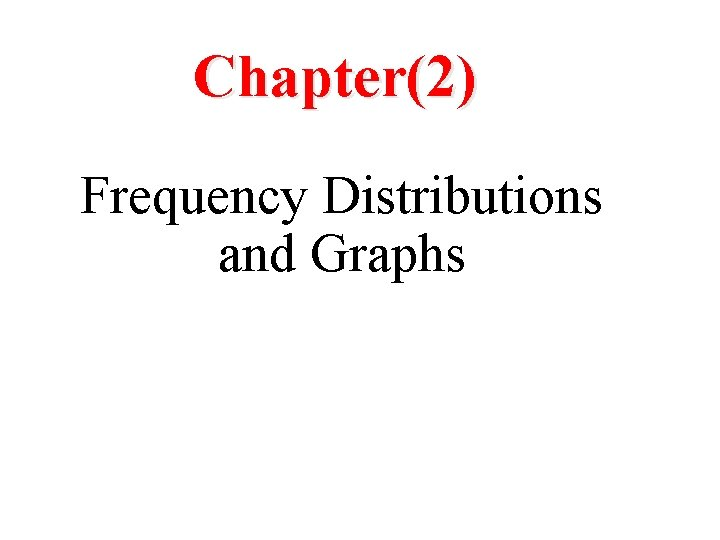Chapter(2) Frequency Distributions and Graphs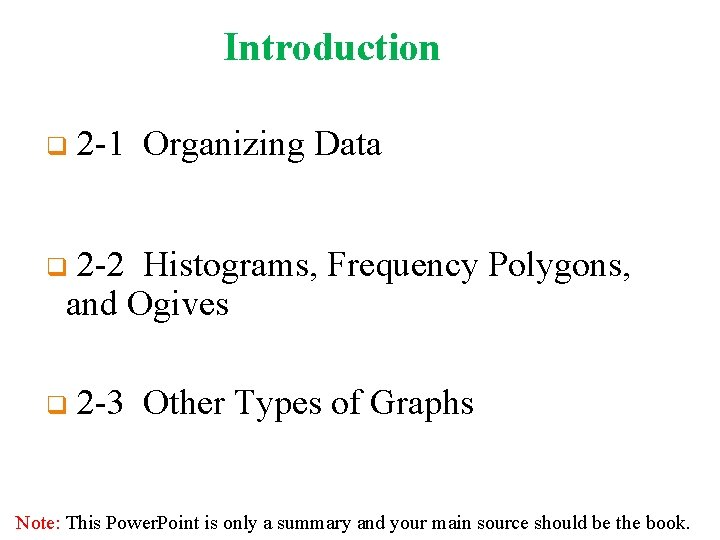Introduction q 2 -1 Organizing Data 2 -2 Histograms, Frequency Polygons, and Ogives q q 2 -3 Other Types of Graphs Note: This Power. Point is only a summary and your main source should be the book.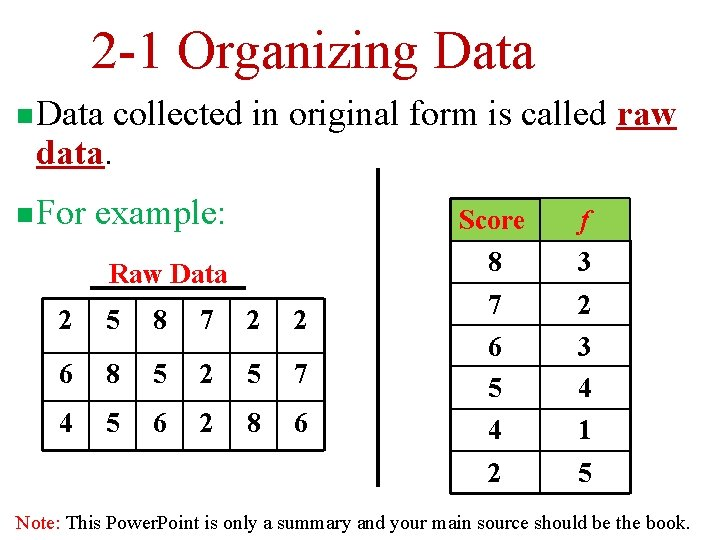2 -1 Organizing Data n Data collected in original form is called raw data. n For example: Score f Raw Data 8 3 7 2 3 4 2 5 8 7 2 2 6 8 5 2 5 7 6 5 4 5 6 2 8 6 4 1 2 5 Note: This Power. Point is only a summary and your main source should be the book.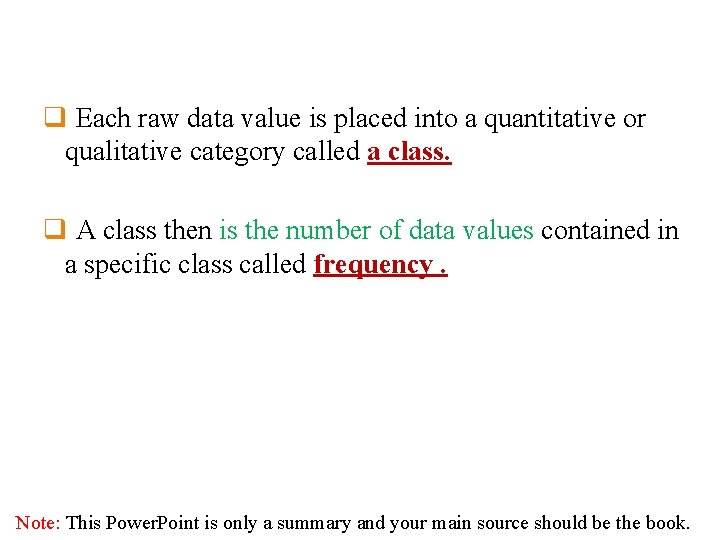q Each raw data value is placed into a quantitative or qualitative category called a class. q A class then is the number of data values contained in a specific class called frequency. Note: This Power. Point is only a summary and your main source should be the book.n. A frequency distribution is the organization of raw data in table form, using classes and frequencies. Two types of frequency distribution Categorical Frequency Distributions Used for data that can be placed in specific categories (nominal or ordinal level data). Grouped Frequency Distributions When the range of the data is large data is grouped into classes that are more than one unit in width Note: This Power. Point is only a summary and your main source should be the book.types of frequency distribution Categorical Frequency Distributions Used for data that can be placed in specific categories (nominal or ordinal level data). Grouped Frequency Distributions When the range of the data is large data is grouped into classes that are more than one unit in width Ungrouped Frequency Distributions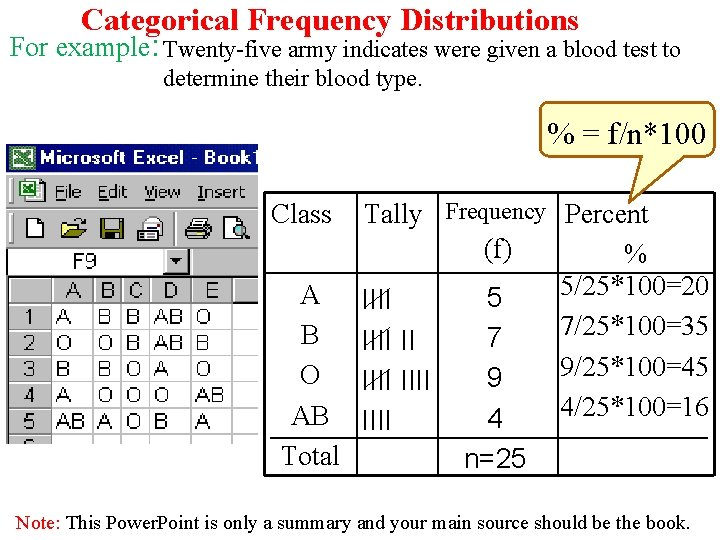Categorical Frequency Distributions For example: Twenty-five army indicates were given a blood test to determine their blood type. % = f/n*100 Tally Frequency Percent (f) % 5/25*100=20 A IIII 5 7/25*100=35 B IIII II 7 9/25*100=45 O IIII 9 4/25*100=16 AB IIII 4 Total n=25 Class Note: This Power. Point is only a summary and your main source should be the book.Grouped Frequency Distributions q Class limits q Lower class limit q. Upper class limit Upper class Lower class First class second class q. Class boundaries q. Upper class boundaries q. Lower class boundaries Class limits 24 -30 31 -37 Class boundaries 23. 5 -30. 5 -37. 5 Tally Frequency /// / 3 1 38 -44 45 -51 52 -58 59 -65 37. 5 -44. 5 -51. 5 -58. 5 -65. 5 //// / / 5 9 6 1 Note: This Power. Point is only a summary and your main source should be the book.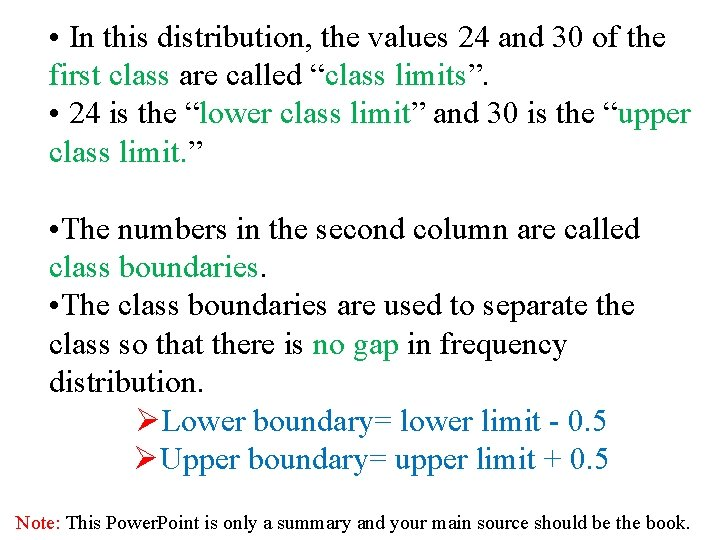• In this distribution, the values 24 and 30 of the first class are called “class limits”. • 24 is the “lower class limit” and 30 is the “upper class limit. ” • The numbers in the second column are called class boundaries. • The class boundaries are used to separate the class so that there is no gap in frequency distribution. ØLower boundary= lower limit - 0. 5 ØUpper boundary= upper limit + 0. 5 Note: This Power. Point is only a summary and your main source should be the book.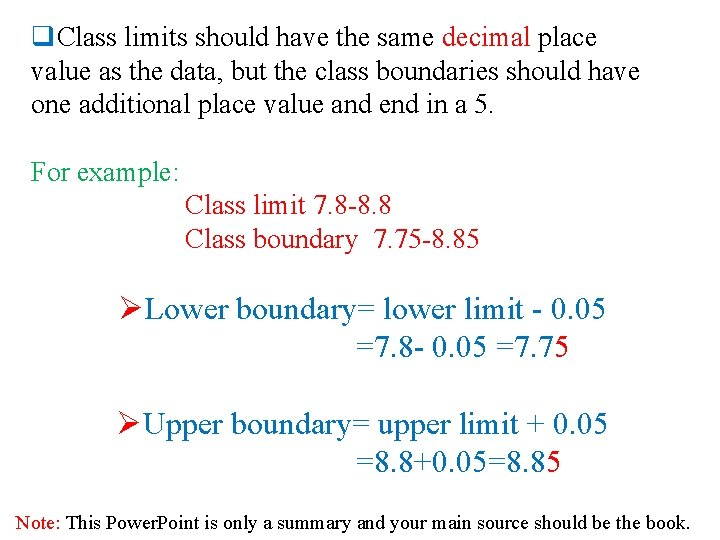q. Class limits should have the same decimal place value as the data, but the class boundaries should have one additional place value and end in a 5. For example: Class limit 7. 8 -8. 8 Class boundary 7. 75 -8. 85 ØLower boundary= lower limit - 0. 05 =7. 8 - 0. 05 =7. 75 ØUpper boundary= upper limit + 0. 05 =8. 8+0. 05=8. 85 Note: This Power. Point is only a summary and your main source should be the book.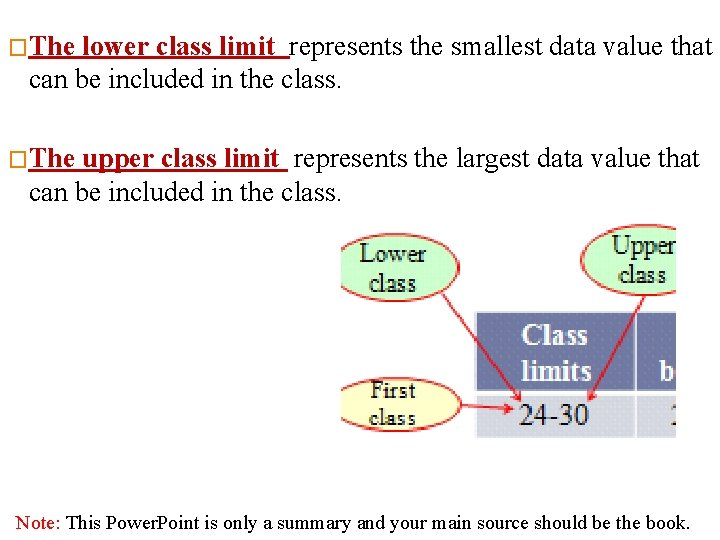�The lower class limit represents the smallest data value that can be included in the class. �The upper class limit represents the largest data value that can be included in the class. Note: This Power. Point is only a summary and your main source should be the book.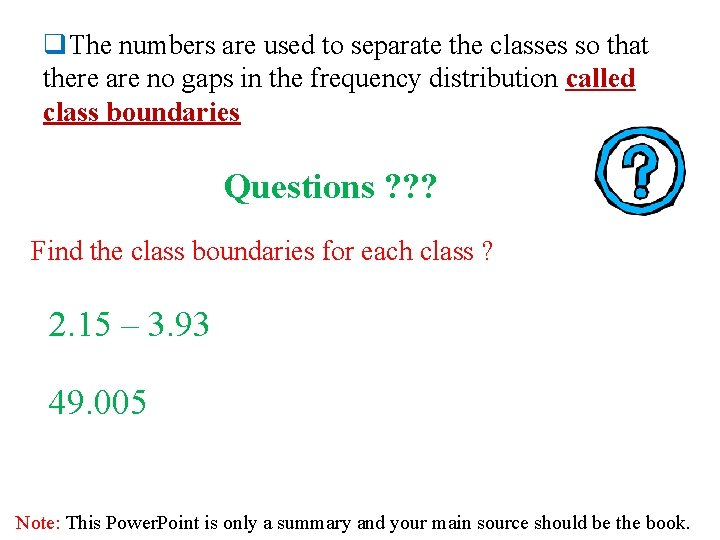q. The numbers are used to separate the classes so that there are no gaps in the frequency distribution called class boundaries Questions ? ? ? Find the class boundaries for each class ? 2. 15 – 3. 93 49. 005 Note: This Power. Point is only a summary and your main source should be the book.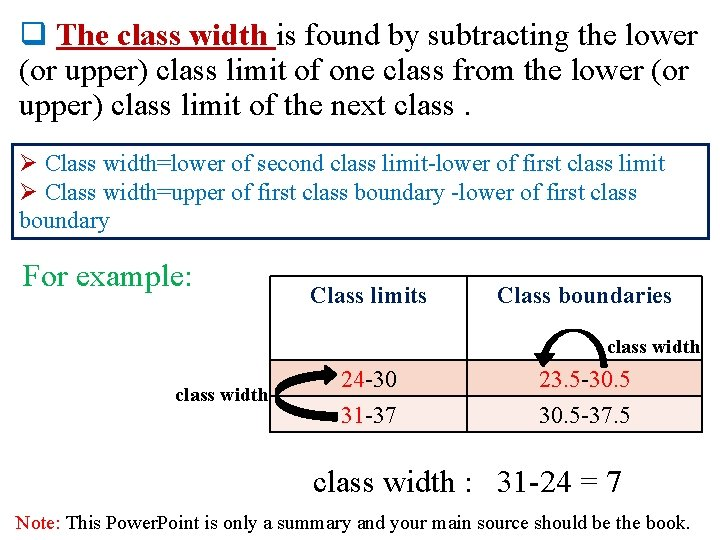q The class width is found by subtracting the lower (or upper) class limit of one class from the lower (or upper) class limit of the next class. Ø Class width=lower of second class limit-lower of first class limit Ø Class width=upper of first class boundary -lower of first class boundary For example: Class limits Class boundaries class width 24 -30 31 -37 23. 5 -30. 5 -37. 5 class width : 31 -24 = 7 Note: This Power. Point is only a summary and your main source should be the book.q. The class midpoint Xm is found by adding the lower and upper class limit (or boundary) and dividing by 2. Xm = Or Xm = For example : Note: This Power. Point is only a summary and your main source should be the book.Rules for Classes in Grouped Frequency Distributions 1. 2. 3. There should be 5 -20 classes. The class width should be an odd number. The classes must be mutually exclusive. Age 10 -20 20 -30 30 -40 Better way to construct a frequency distribution 21 -31 32 -42 40 -50 43 -53 50 -60 54 -64 . The classes must be continuous. 5. The classes must be exhaustive. 6. The classes must be equal in width (except in open-ended distributions). 4 Note: This Power. Point is only a summary and your main source should be the book.Constructing a Grouped Frequency Distribution 1 - The following data represent the record high temperatures for each of the 50 states. Construct a grouped frequency distribution for the data using 7 classes. 112 110 107 116 120 100 118 112 108 113 127 114 110 120 116 115 121 117 134 118 113 105 118 122 117 120 110 105 114 118 119 118 110 114 122 111 112 109 105 106 104 112 109 110 111 114 Note: This Power. Point is only a summary and your main source should be the book.STEP 1 Determine the classes. Find the class width by dividing the range by the number of classes 7. Range = High – Low = 134 – 100 = 34 Rou nd u p Width = Range/7 = 34/7 ≈ 4. 9=5 Note: This Power. Point is only a summary and your main source should be the book.Step 2 : Tally the data. Step 3 : Find the frequencies. Class Limits Class Boundaries Frequency 100 - 104 105 - 109 110 - 114 115 - 119 120 - 124 125 - 129 130 - 134 99. 5 - 104. 5 - 109. 5 - 114. 5 - 119. 5 - 124. 5 - 129. 5 - 134. 5 2 8 18 13 7 1 1 Cumulative Frequency 0 2 10 28 41 48 49 50 Note: This Power. Point is only a summary and your main source should be the book.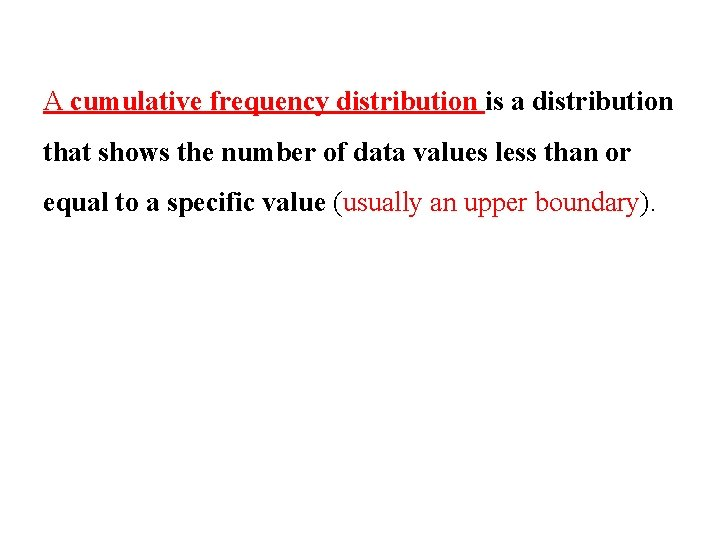A cumulative frequency distribution is a distribution that shows the number of data values less than or equal to a specific value (usually an upper boundary).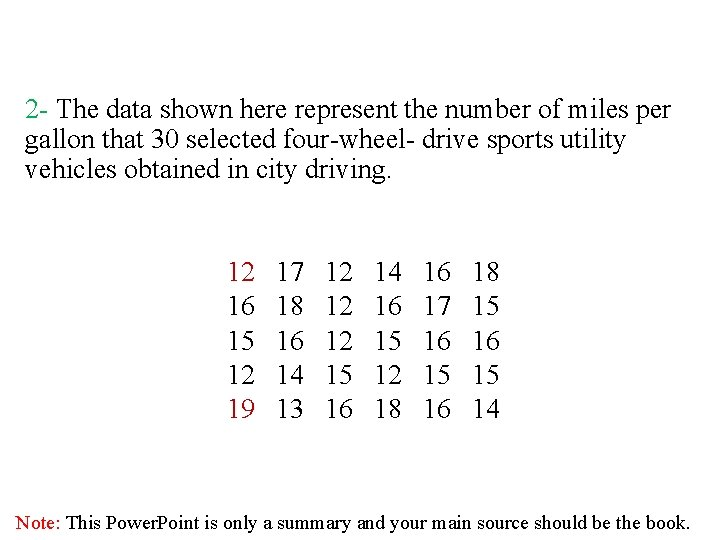2 - The data shown here represent the number of miles per gallon that 30 selected four-wheel- drive sports utility vehicles obtained in city driving. 12 16 15 12 19 17 18 16 14 13 12 12 12 15 16 14 16 15 12 18 16 17 16 15 16 18 15 16 15 14 Note: This Power. Point is only a summary and your main source should be the book.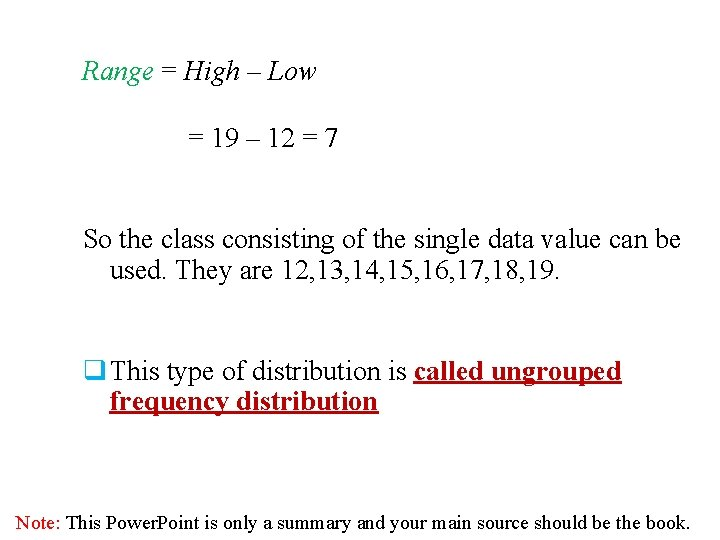Range = High – Low = 19 – 12 = 7 So the class consisting of the single data value can be used. They are 12, 13, 14, 15, 16, 17, 18, 19. q This type of distribution is called ungrouped frequency distribution Note: This Power. Point is only a summary and your main source should be the book.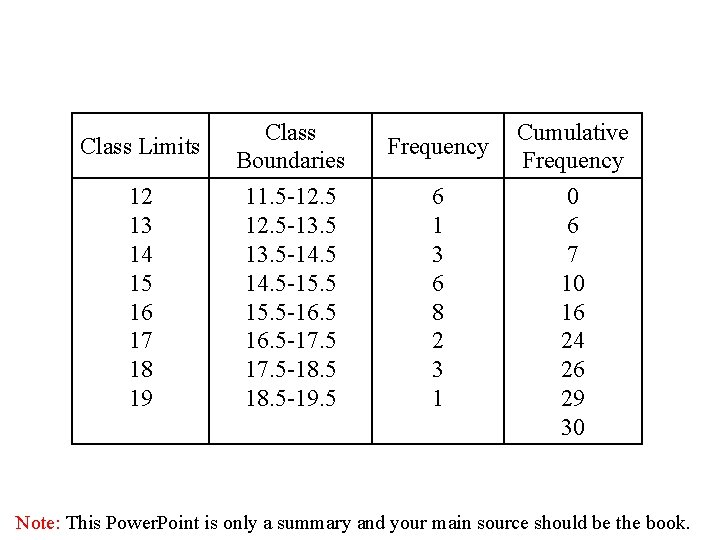Class Limits 12 13 14 15 16 17 18 19 Class Boundaries 11. 5 -12. 5 -13. 5 -14. 5 -15. 5 -16. 5 -17. 5 -18. 5 -19. 5 Frequency 6 1 3 6 8 2 3 1 Cumulative Frequency 0 6 7 10 16 24 26 29 30 Note: This Power. Point is only a summary and your main source should be the book.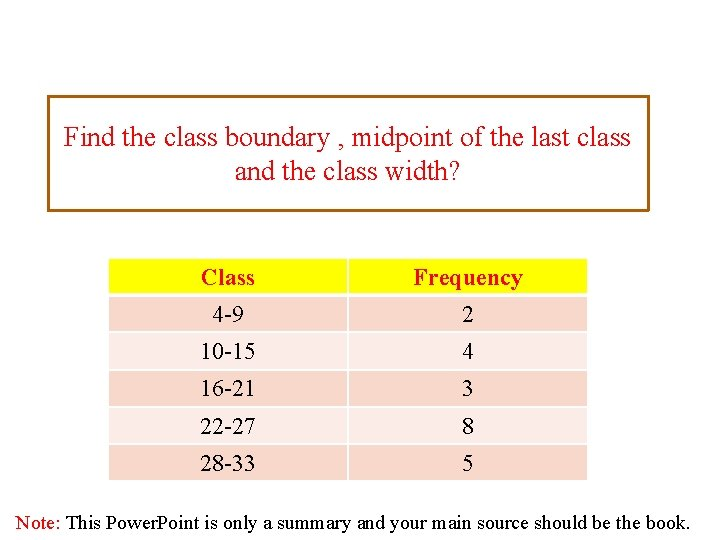Find the class boundary , midpoint of the last class and the class width? Class Frequency 4 -9 2 10 -15 4 16 -21 3 22 -27 8 28 -33 5 Note: This Power. Point is only a summary and your main source should be the book.Solution Xm Class Boundaries 4 -9 3. 5 – 9. 5 10 -15 9. 5 -15. 5 16 -21 15. 5 -21. 5 22 -27 21. 5 -27. 5 28 -33 27. 5 -33. 5 = Class width= 10 - 4 = 6 Note: This Power. Point is only a summary and your main source should be the book.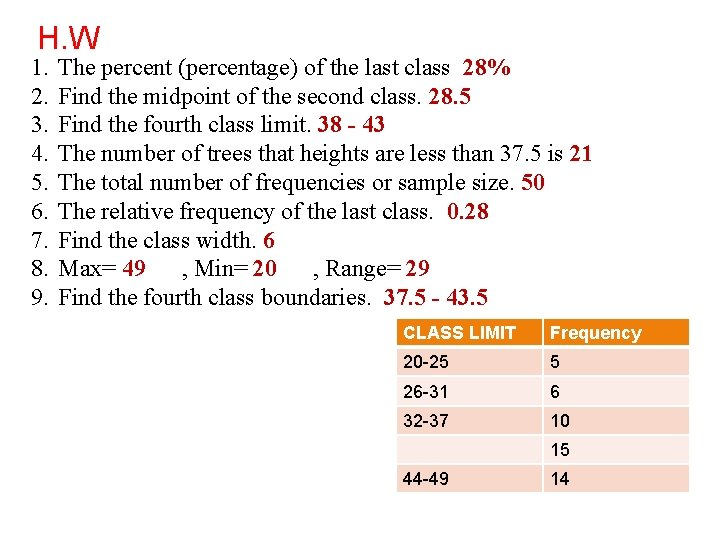H. W 1. 2. 3. 4. 5. 6. 7. 8. 9. The percent (percentage) of the last class 28% Find the midpoint of the second class. 28. 5 Find the fourth class limit. 38 - 43 The number of trees that heights are less than 37. 5 is 21 The total number of frequencies or sample size. 50 The relative frequency of the last class. 0. 28 Find the class width. 6 Max= 49 , Min= 20 , Range= 29 Find the fourth class boundaries. 37. 5 - 43. 5 CLASS LIMIT Frequency 20 -25 5 26 -31 6 32 -37 10 15 44 -49 14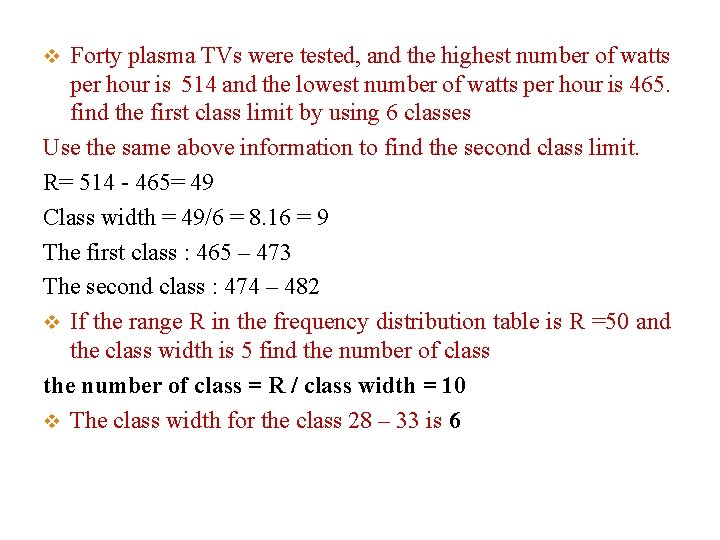Forty plasma TVs were tested, and the highest number of watts per hour is 514 and the lowest number of watts per hour is 465. find the first class limit by using 6 classes Use the same above information to find the second class limit. R= 514 - 465= 49 Class width = 49/6 = 8. 16 = 9 The first class : 465 – 473 The second class : 474 – 482 v If the range R in the frequency distribution table is R =50 and the class width is 5 find the number of class = R / class width = 10 v The class width for the class 28 – 33 is 6 vHistograms, Frequency Polygons, and Ogives Note: This Power. Point is only a summary and your main source should be the book.For Continuous Data The three most commonly used graphs in research are as follows: 1. The histogram 2. The frequency polygon 3. The cumulative frequency graph, or Ogive Note: This Power. Point is only a summary and your main source should be the book.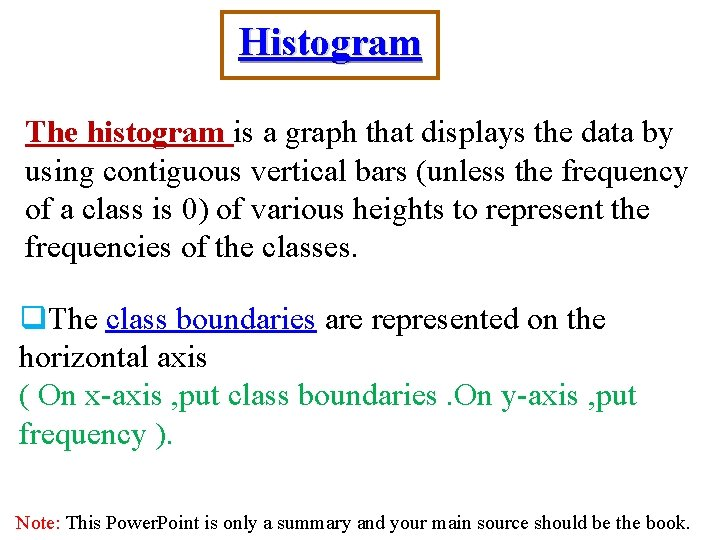Histogram The histogram is a graph that displays the data by using contiguous vertical bars (unless the frequency of a class is 0) of various heights to represent the frequencies of the classes. q. The class boundaries are represented on the horizontal axis ( On x-axis , put class boundaries. On y-axis , put frequency ). Note: This Power. Point is only a summary and your main source should be the book.Example 2 -4: Construct a histogram to represent the data for the record high temperatures for each of the 50 states (see Example 2– 2 for the data). Class Limits Class Boundaries Frequency 100 - 104 105 - 109 110 - 114 115 - 119 120 - 124 125 - 129 130 - 134 99. 5 - 104. 5 - 109. 5 - 114. 5 - 119. 5 - 124. 5 - 129. 5 - 134. 5 2 8 18 13 7 1 1 Note: This Power. Point is only a summary and your main source should be the book.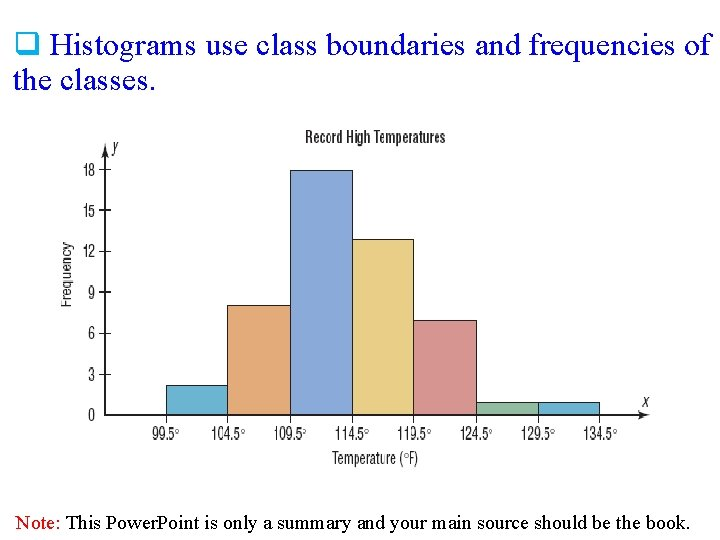q Histograms use class boundaries and frequencies of the classes. Note: This Power. Point is only a summary and your main source should be the book.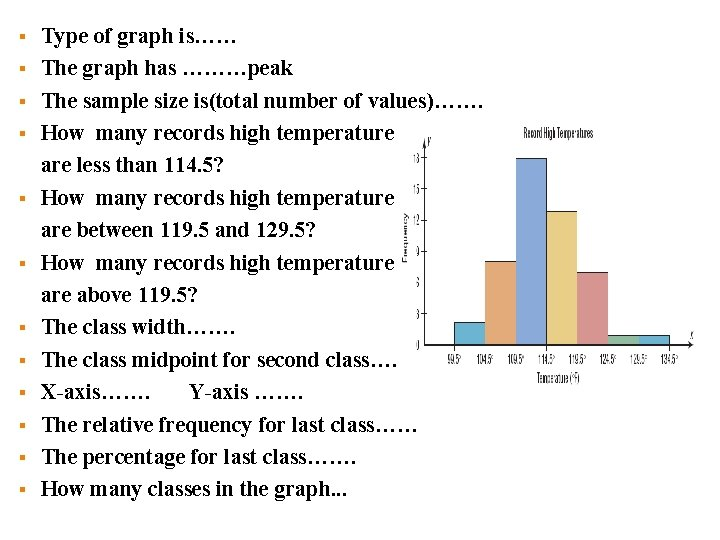§ § § Type of graph is…… The graph has ………peak The sample size is(total number of values)……. How many records high temperature are less than 114. 5? How many records high temperature are between 119. 5 and 129. 5? How many records high temperature above 119. 5? The class width……. The class midpoint for second class…. X-axis……. Y-axis ……. The relative frequency for last class…… The percentage for last class……. How many classes in the graph. . .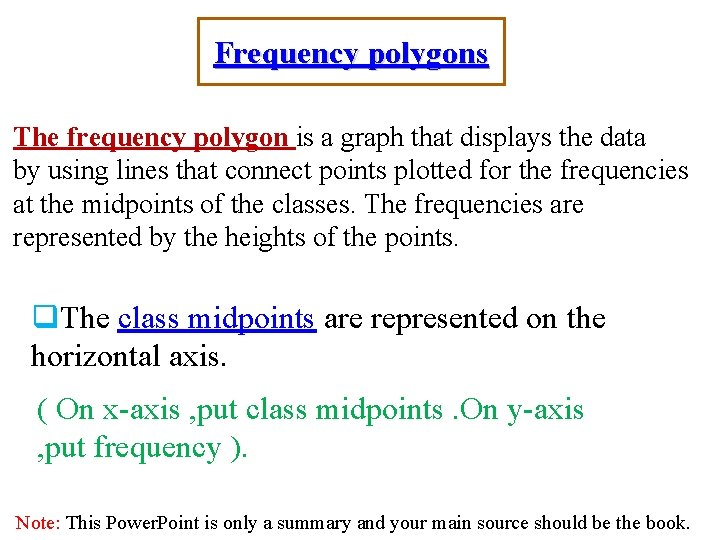Frequency polygons The frequency polygon is a graph that displays the data by using lines that connect points plotted for the frequencies at the midpoints of the classes. The frequencies are represented by the heights of the points. q. The class midpoints are represented on the horizontal axis. ( On x-axis , put class midpoints. On y-axis , put frequency ). Note: This Power. Point is only a summary and your main source should be the book.Example 2 -5: Construct a frequency polygon to represent the data for the record high temperatures for each of the 50 states (see Example 2– 2 for the data). Class Limits Class Midpoints Frequency 100 - 104 105 - 109 110 - 114 115 - 119 120 - 124 125 - 129 130 - 134 102 107 112 117 122 127 132 2 8 18 13 7 1 1 Note: This Power. Point is only a summary and your main source should be the book.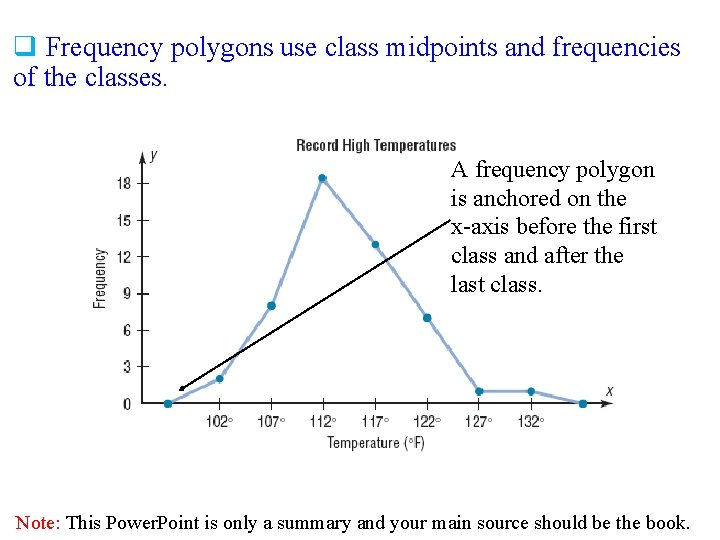q Frequency polygons use class midpoints and frequencies of the classes. A frequency polygon is anchored on the x-axis before the first class and after the last class. Note: This Power. Point is only a summary and your main source should be the book.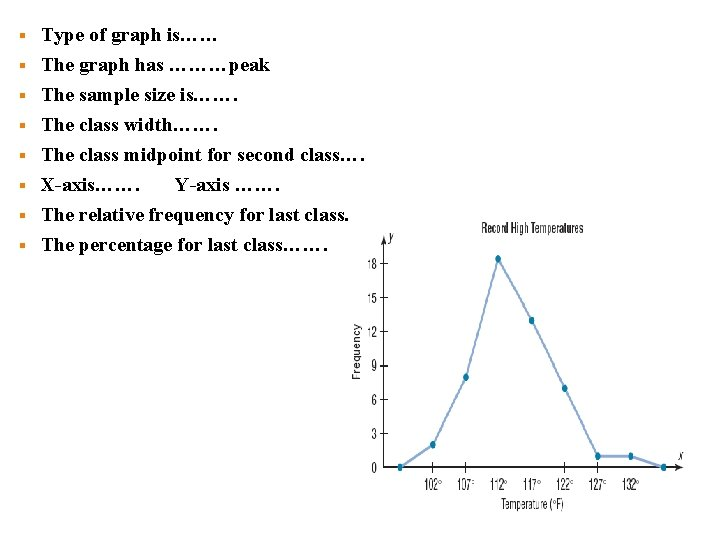§ § § § Type of graph is…… The graph has ………peak The sample size is……. The class width……. The class midpoint for second class…. X-axis……. Y-axis ……. The relative frequency for last class…… The percentage for last class…….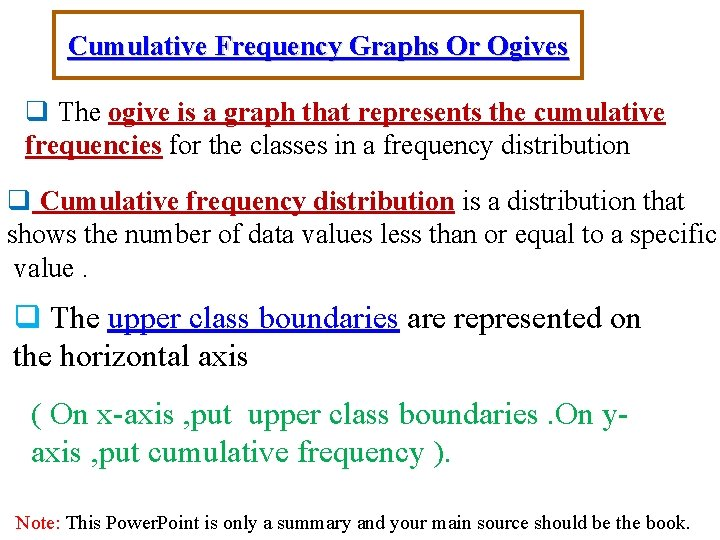Cumulative Frequency Graphs Or Ogives q The ogive is a graph that represents the cumulative frequencies for the classes in a frequency distribution q Cumulative frequency distribution is a distribution that shows the number of data values less than or equal to a specific value. q The upper class boundaries are represented on the horizontal axis ( On x-axis , put upper class boundaries. On yaxis , put cumulative frequency ). Note: This Power. Point is only a summary and your main source should be the book.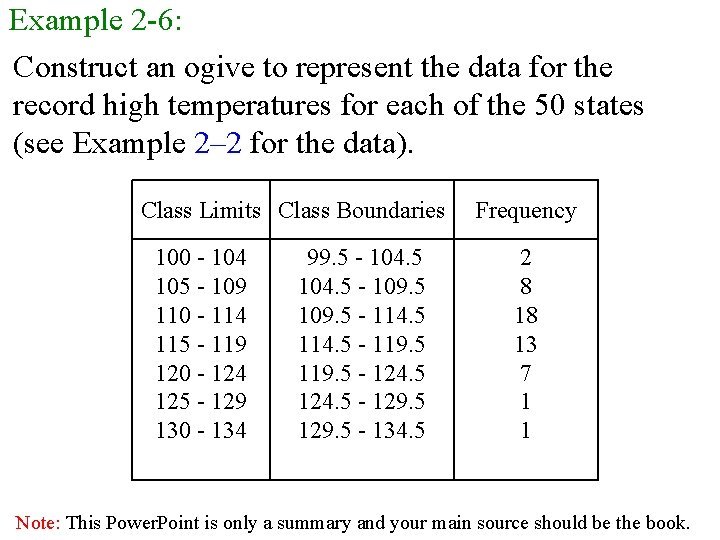Example 2 -6: Construct an ogive to represent the data for the record high temperatures for each of the 50 states (see Example 2– 2 for the data). Class Limits Class Boundaries 100 - 104 105 - 109 110 - 114 115 - 119 120 - 124 125 - 129 130 - 134 99. 5 - 104. 5 - 109. 5 - 114. 5 - 119. 5 - 124. 5 - 129. 5 - 134. 5 Frequency 2 8 18 13 7 1 1 Note: This Power. Point is only a summary and your main source should be the book.Class Limits Class Boundaries Frequency 100 - 104 105 - 109 110 - 114 115 - 119 120 - 124 125 - 129 130 - 134 99. 5 - 104. 5 - 109. 5 - 114. 5 - 119. 5 - 124. 5 - 129. 5 - 134. 5 2 8 18 13 7 1 1 Class Boundaries Cumulative Frequency Less than 99. 5 Less than 104. 5 Less than 109. 5 Less than 114. 5 Less than 119. 5 Less than 124. 5 Less than 129. 5 Less than 134. 5 0 2 10 28 41 48 49 50 Note: This Power. Point is only a summary and your main source should be the book.q Cumulative frequency is the sum of the frequencies accumulated up to the upper boundary of a class in the distortion.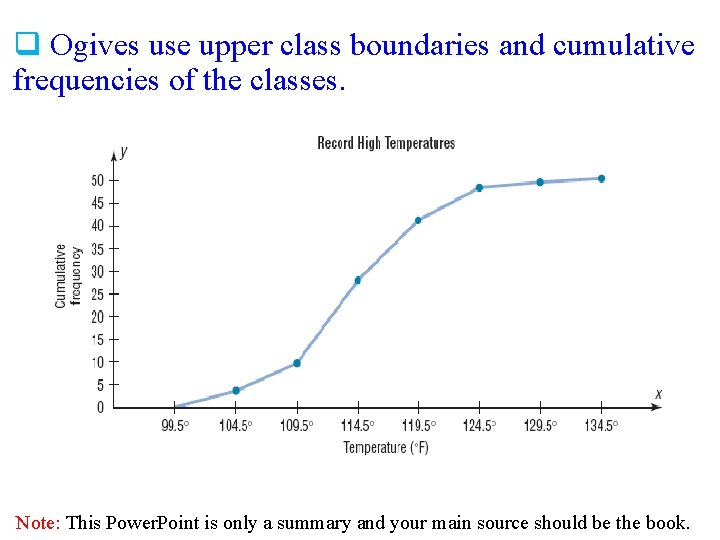q Ogives use upper class boundaries and cumulative frequencies of the classes. Note: This Power. Point is only a summary and your main source should be the book.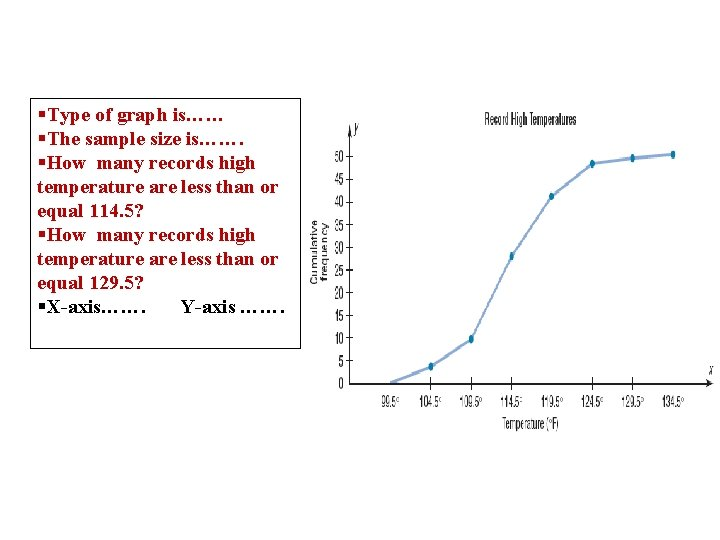§Type of graph is…… §The sample size is……. §How many records high temperature are less than or equal 114. 5? §How many records high temperature are less than or equal 129. 5? §X-axis……. Y-axis …….The Relative Frequency q The distribution using proportions instead f raw data as frequencies called relative frequency. Note: This Power. Point is only a summary and your main source should be the book.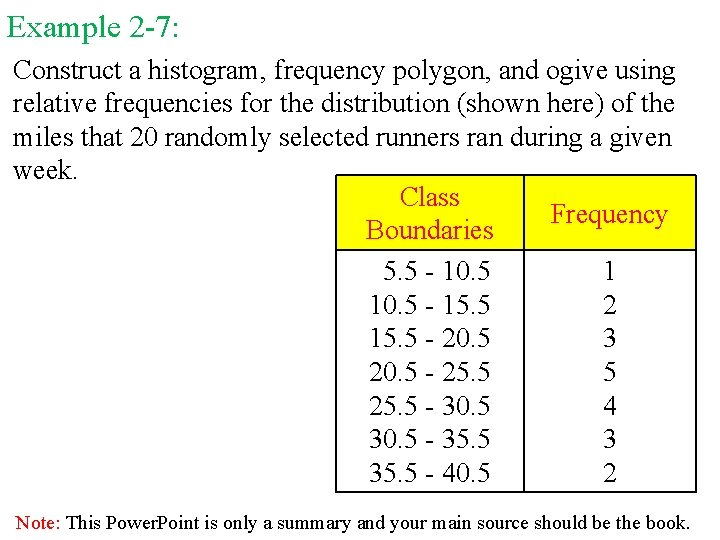Example 2 -7: Construct a histogram, frequency polygon, and ogive using relative frequencies for the distribution (shown here) of the miles that 20 randomly selected runners ran during a given week. Class Frequency Boundaries 5. 5 - 10. 5 1 10. 5 - 15. 5 2 15. 5 - 20. 5 3 20. 5 - 25. 5 5 25. 5 - 30. 5 4 30. 5 - 35. 5 3 35. 5 - 40. 5 2 Note: This Power. Point is only a summary and your main source should be the book.Histograms The following is a frequency distribution of miles run per week by 20 selected runners. Class Boundaries Frequency (f) 5. 5 - 10. 5 - 15. 5 - 20. 5 - 25. 5 - 30. 5 - 35. 5 - 40. 5 1 2 3 5 4 3 2 f = 20 Relative Frequency 1/20 = 0. 05 2/20 = 0. 10 3/20 = 0. 15 5/20 = 0. 25 4/20 = 0. 20 3/20 = 0. 15 2/20 = 0. 10 rf = 1. 00 The sum of the relative frequencies will always be 1 Note: This Power. Point is only a summary and your main source should be the book.q. Use the class boundaries and the relative frequencies of the classes. Note: This Power. Point is only a summary and your main source should be the book.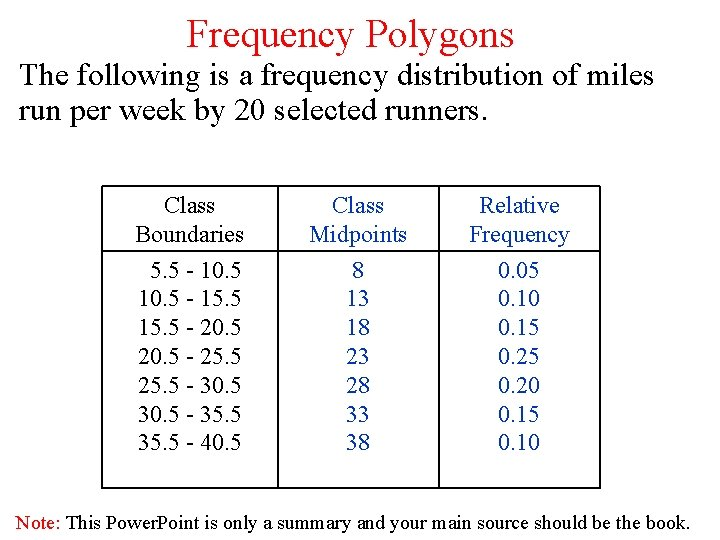Frequency Polygons The following is a frequency distribution of miles run per week by 20 selected runners. Class Boundaries Class Midpoints Relative Frequency 5. 5 - 10. 5 - 15. 5 - 20. 5 - 25. 5 - 30. 5 - 35. 5 - 40. 5 8 13 18 23 28 33 38 0. 05 0. 10 0. 15 0. 20 0. 15 0. 10 Note: This Power. Point is only a summary and your main source should be the book.q. Use the class midpoints and the relative frequencies of the classes. Note: This Power. Point is only a summary and your main source should be the book.Ogives The following is a frequency distribution of miles run per week by 20 selected runners. Class Boundaries 5. 5 - 10. 5 - 15. 5 - 20. 5 - 25. 5 - 30. 5 - 35. 5 - 40. 5 Frequency Cumulative Frequency 1 2 3 5 4 3 2 f = 20 0 1 3 6 11 15 18 20 Cum. Rel. Frequency 0 0 1/20 = 0. 05 3/20 = 0. 15 6/20 = 0. 30 11/20 = 0. 55 15/20 = 0. 75 18/20 = 0. 90 20/20 = 1. 00 Note: This Power. Point is only a summary and your main source should be the book.q Ogives use upper class boundaries and cumulative frequencies of the classes. Class Boundaries Cum. Rel. Frequency Less than 5. 5 Less than 10. 5 Less than 15. 5 Less than 20. 5 Less than 25. 5 Less than 30. 5 Less than 35. 5 Less than 40. 5 0 0. 05 0. 15 0. 30 0. 55 0. 75 0. 90 1. 00 Note: This Power. Point is only a summary and your main source should be the book.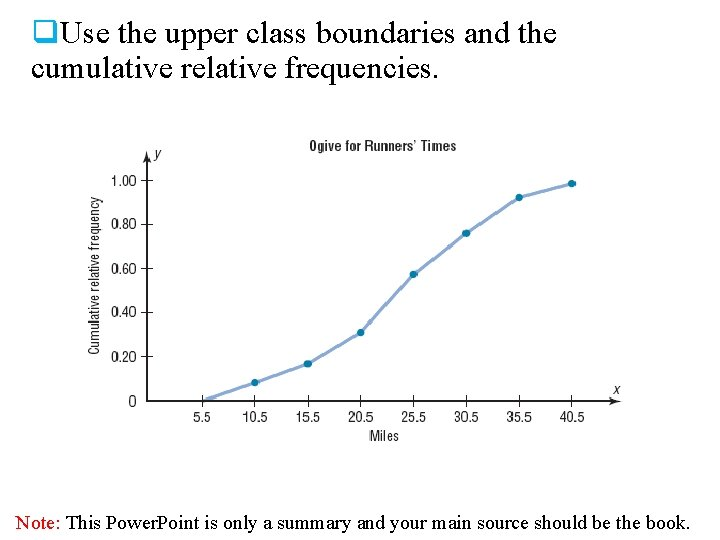q. Use the upper class boundaries and the cumulative relative frequencies. Note: This Power. Point is only a summary and your main source should be the book.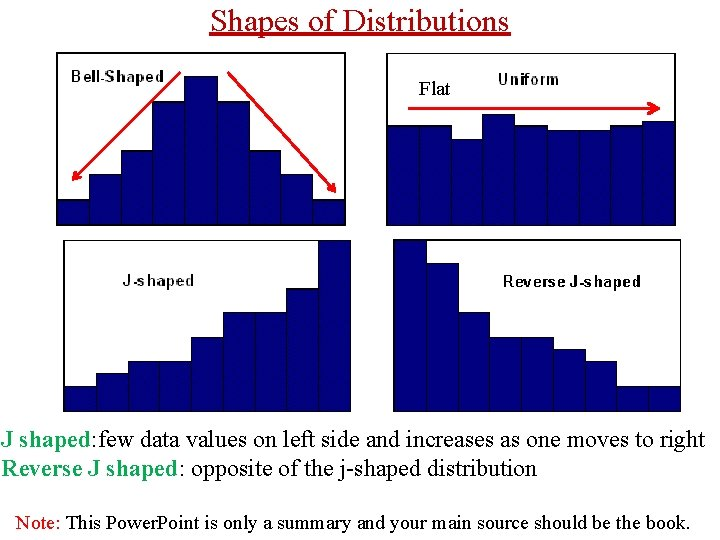Shapes of Distributions Flat J shaped: few data values on left side and increases as one moves to right Reverse J shaped: opposite of the j-shaped distribution Note: This Power. Point is only a summary and your main source should be the book.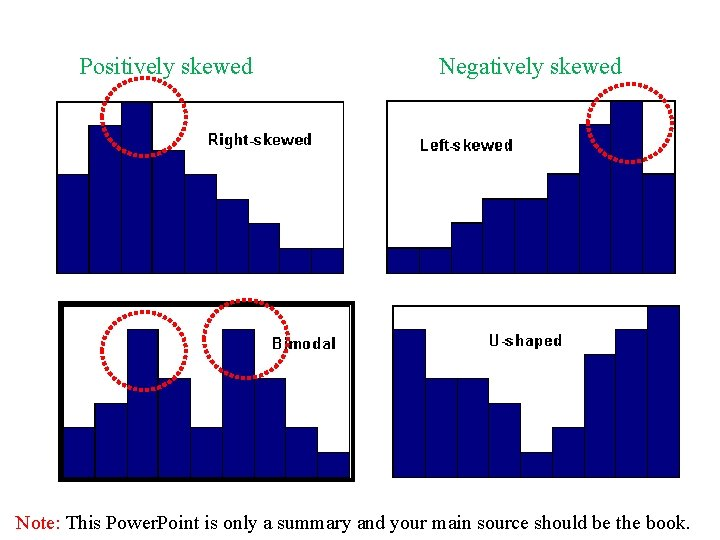Positively skewed Negatively skewed Note: This Power. Point is only a summary and your main source should be the book.Other Types of Graphs Several other types of graphs are often used in statistics. We will discuss three other types of graphs as follows: 1. A bar graph 2. A Pareto chart 3. The Time series graph 4. The Pie graph Note: This Power. Point is only a summary and your main source should be the book.q. A bar graph represents the data by using vertical or horizontal bars whose heights or lengths represent the frequencies of the data. When the data are qualitative or categorical , bar graphs can be used. Page (76) Note: This Power. Point is only a summary and your main source should be the book.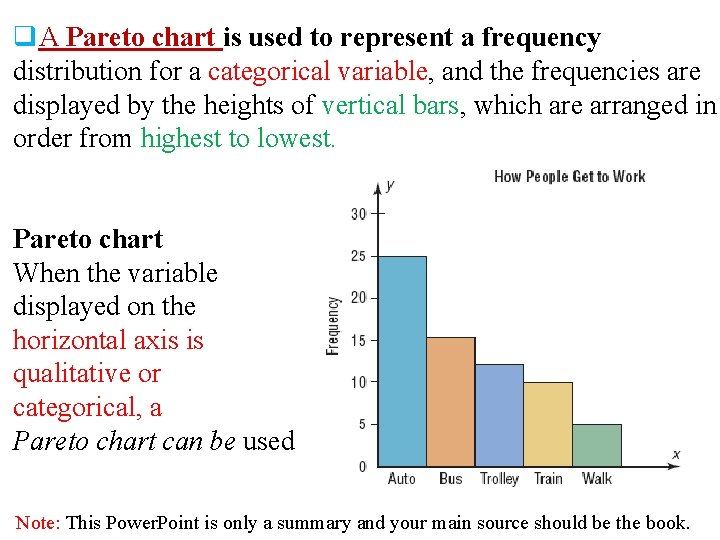q. A Pareto chart is used to represent a frequency distribution for a categorical variable, and the frequencies are displayed by the heights of vertical bars, which are arranged in order from highest to lowest. Pareto chart When the variable displayed on the horizontal axis is qualitative or categorical, a Pareto chart can be used Note: This Power. Point is only a summary and your main source should be the book.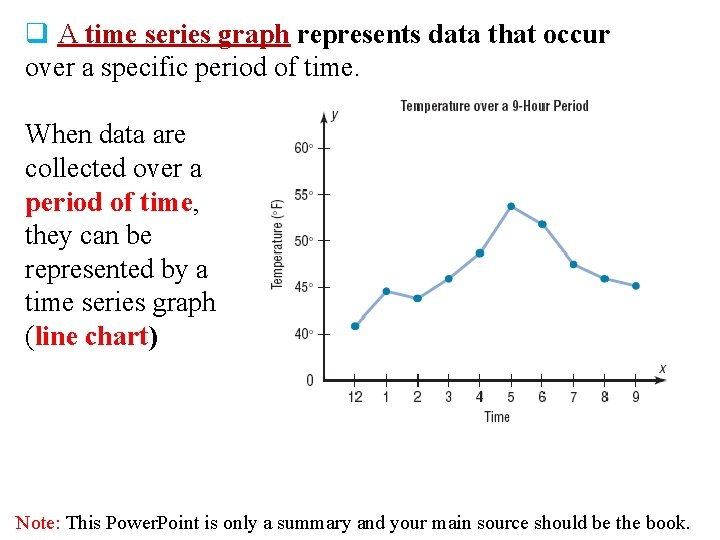q A time series graph represents data that occur over a specific period of time. When data are collected over a period of time, they can be represented by a time series graph (line chart) Note: This Power. Point is only a summary and your main source should be the book.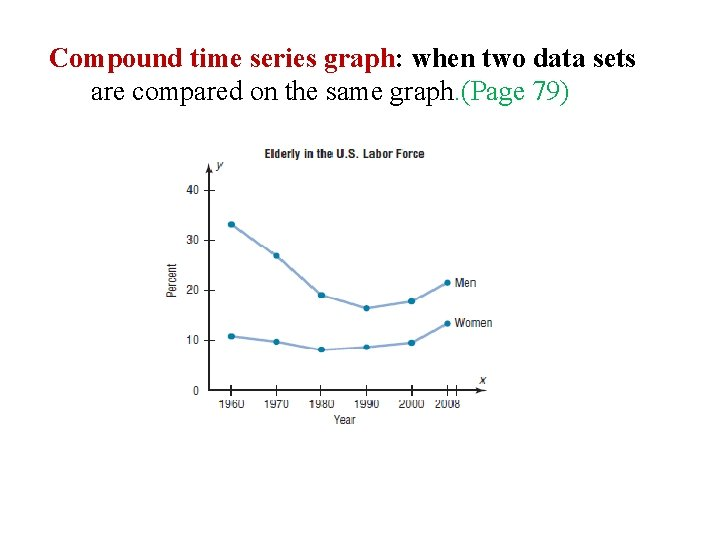Compound time series graph: when two data sets are compared on the same graph. (Page 79)q. A pie graph is a circle that is divided into sections or wedges according to the percentage of frequencies in each category of the distribution. q. The purpose of the pie graph is to show the relationship of the parts to the whole by visually comparing the sizes of the sections. q. Percentages or proportions can be used. q. The variable is nominal or categorical. Note: This Power. Point is only a summary and your main source should be the book.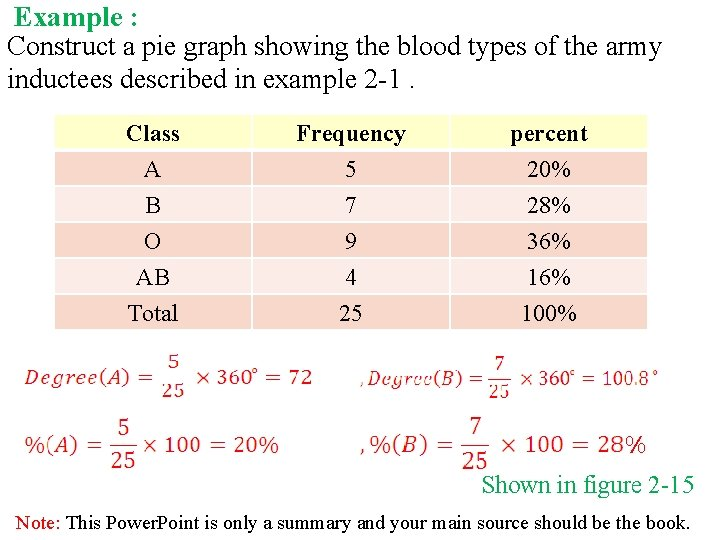Example : Construct a pie graph showing the blood types of the army inductees described in example 2 -1. Class A B O Frequency 5 7 9 percent 20% 28% 36% AB Total 4 25 16% 100% % Shown in figure 2 -15 Note: This Power. Point is only a summary and your main source should be the book.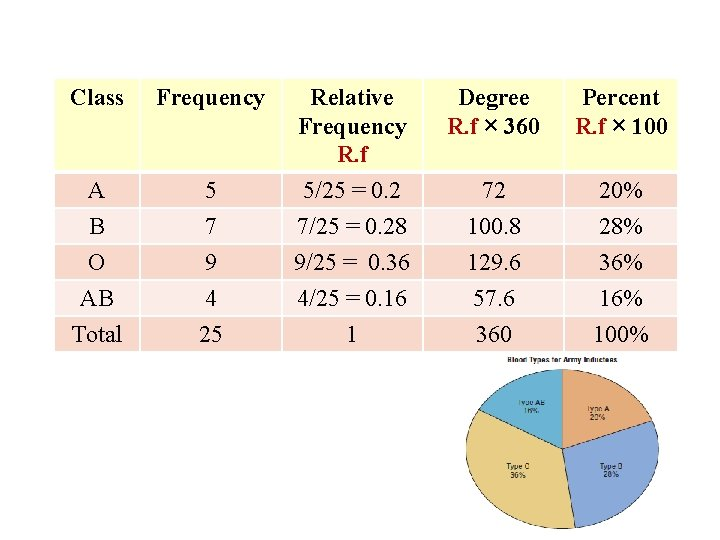Class Frequency Degree R. f × 360 Percent R. f × 100 5 Relative Frequency R. f 5/25 = 0. 2 A 72 20% B O AB Total 7 9 4 25 7/25 = 0. 28 9/25 = 0. 36 4/25 = 0. 16 1 100. 8 129. 6 57. 6 360 28% 36% 100%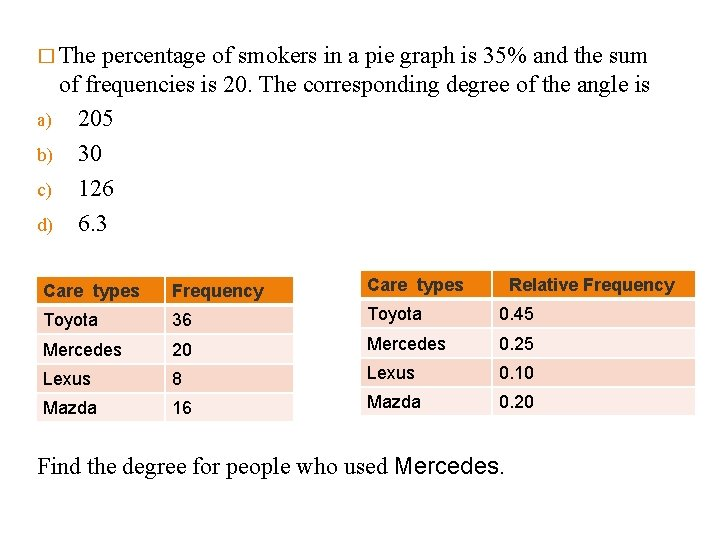� The percentage of smokers in a pie graph is 35% and the sum of frequencies is 20. The corresponding degree of the angle is a) 205 b) 30 c) 126 d) 6. 3 Care types Frequency Care types Relative Frequency Toyota 36 Toyota 0. 45 Mercedes 20 Mercedes 0. 25 Lexus 8 Lexus 0. 10 Mazda 16 Mazda 0. 20 Find the degree for people who used Mercedes.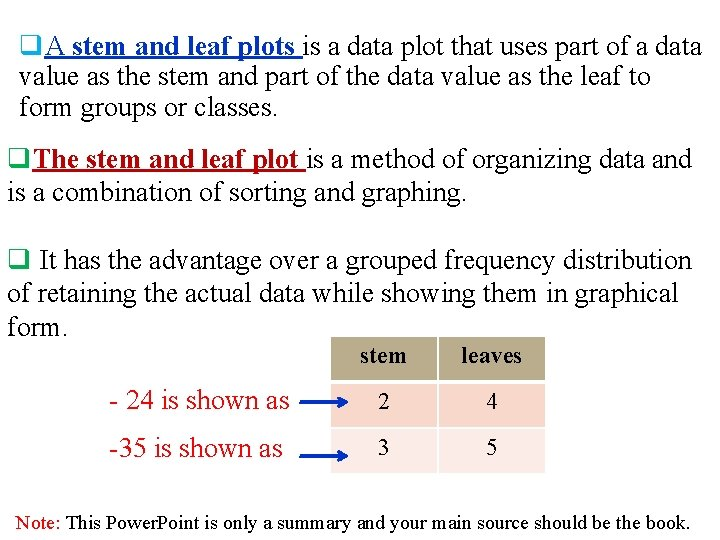q. A stem and leaf plots is a data plot that uses part of a data value as the stem and part of the data value as the leaf to form groups or classes. q. The stem and leaf plot is a method of organizing data and is a combination of sorting and graphing. q It has the advantage over a grouped frequency distribution of retaining the actual data while showing them in graphical form. stem leaves - 24 is shown as 2 4 -35 is shown as 3 5 Note: This Power. Point is only a summary and your main source should be the book.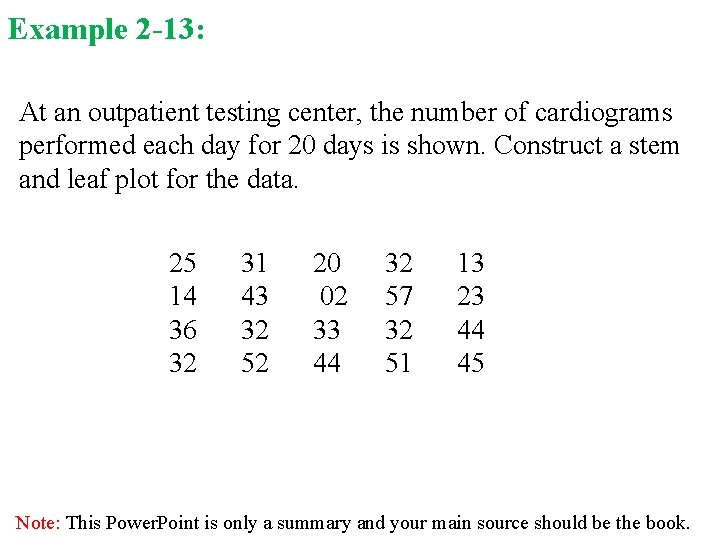Example 2 -13: At an outpatient testing center, the number of cardiograms performed each day for 20 days is shown. Construct a stem and leaf plot for the data. 25 14 36 32 31 43 32 52 20 02 33 44 32 57 32 51 13 23 44 45 Note: This Power. Point is only a summary and your main source should be the book.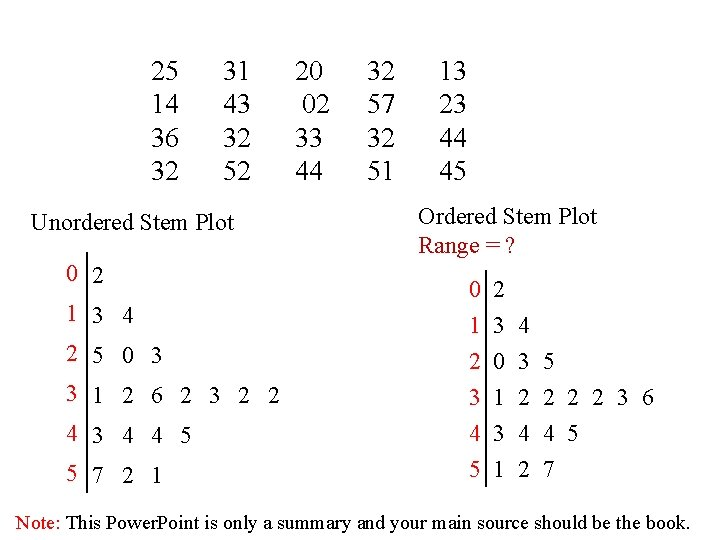25 14 36 32 31 43 32 52 Unordered Stem Plot 0 2 1 3 4 2 5 0 3 3 1 2 6 2 3 2 2 4 3 4 4 5 5 7 2 1 20 02 33 44 32 57 32 51 13 23 44 45 Ordered Stem Plot Range = ? 0 1 2 3 4 0 3 5 1 2 2 3 6 4 3 4 4 5 5 1 2 7 Note: This Power. Point is only a summary and your main source should be the book.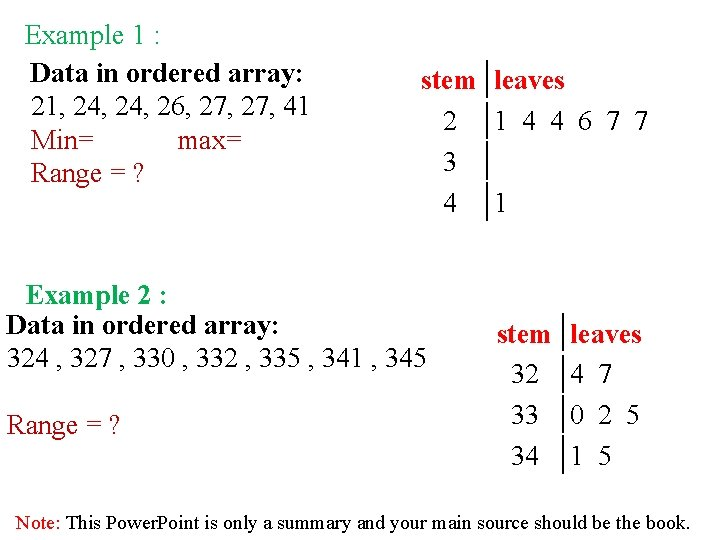Example 1 : Data in ordered array: 21, 24, 26, 27, 41 Min= max= Range = ? stem leaves 2 1 4 4 6 7 7 3 4 1 Example 2 : Data in ordered array: 324 , 327 , 330 , 332 , 335 , 341 , 345 Range = ? stem 32 33 34 leaves 4 7 0 2 5 1 5 Note: This Power. Point is only a summary and your main source should be the book.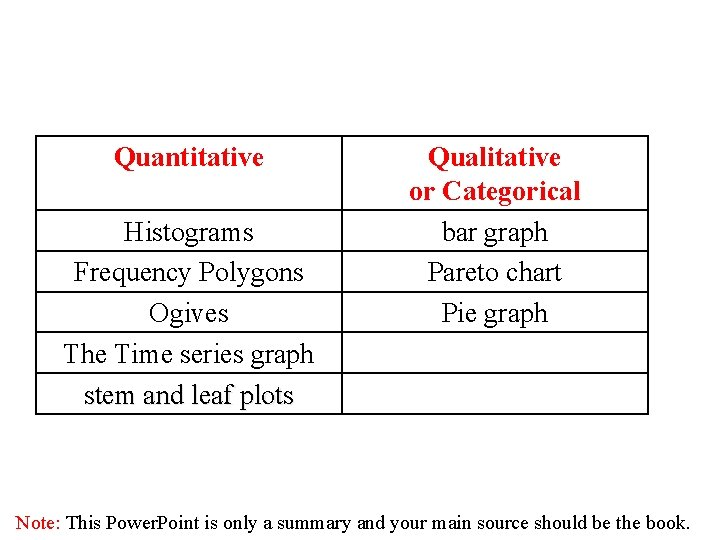Quantitative Histograms Frequency Polygons Ogives The Time series graph stem and leaf plots Qualitative or Categorical bar graph Pareto chart Pie graph Note: This Power. Point is only a summary and your main source should be the book.